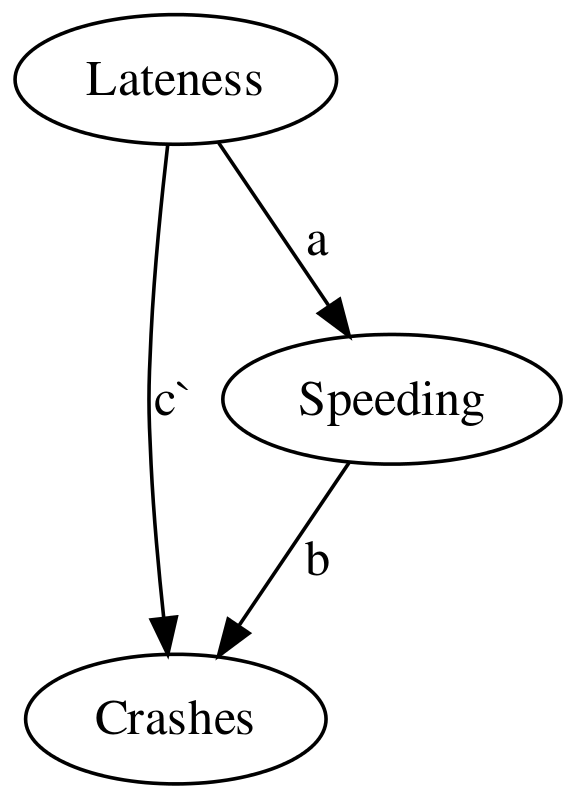## Testing the indirect effect

Baron and Kenny also introduced conventions for labelling some of the coefficients from the regressions described above.

Specifically, the described a as the path from the predictor to the mediator, b as the path from the mediator to the outcome, and c' (c prime) as the path from predictor to outcome, controlling for the mediator. As shown here:

knit_gv("
Lateness -> Crashes[label='c']
Lateness -> Speeding[label=a]
Speeding -> Crashes[label=b]
")Subsequent authors wished to provide a test for whether the path through a and b — the indirect effect — was statistically significant. @preacher_spss_2004 published SPSS macros for computing this indirect effect and providing a non-parametric (bootstrapped) test of this term. The same approach is now implemented in a number of R packages.

The mediation::mediate function accepts the 2nd and 3rd regression models from the ‘Baron and Kenny’ steps, along with arguments which identify which variables are the predictor and the mediator. From this, the function calculates the indirect effect, and the proportion of the total effect mediated. This is accompanied by a bootstrapped standard-error, and asociated p value.

For example, using the models we ran above, we can say:

set.seed(1234)
crashes.mediation <- mediation::mediate(step2, step3, treat = "lateness", mediator = "speed")
summary(crashes.mediation)

Causal Mediation Analysis

Quasi-Bayesian Confidence Intervals

Estimate 95% CI Lower 95% CI Upper p-value
ACME             0.1477       0.0237         0.28   0.018 *
ADE              0.2998       0.1166         0.50  <2e-16 ***
Total Effect     0.4475       0.2293         0.66  <2e-16 ***
Prop. Mediated   0.3295       0.0741         0.60   0.018 *
---
Signif. codes:  0 '***' 0.001 '**' 0.01 '*' 0.05 '.' 0.1 ' ' 1

Sample Size Used: 200

Simulations: 1000 `

From this output, we can see that the indirect effect is statistically significant, and that around hald of the total effect is mediated via speed. Because lateness in iteself is not a plausable cause of a crash, this suggest that other factors (perhaps distraction, inattention) might be important in mediating this residual direct effect.